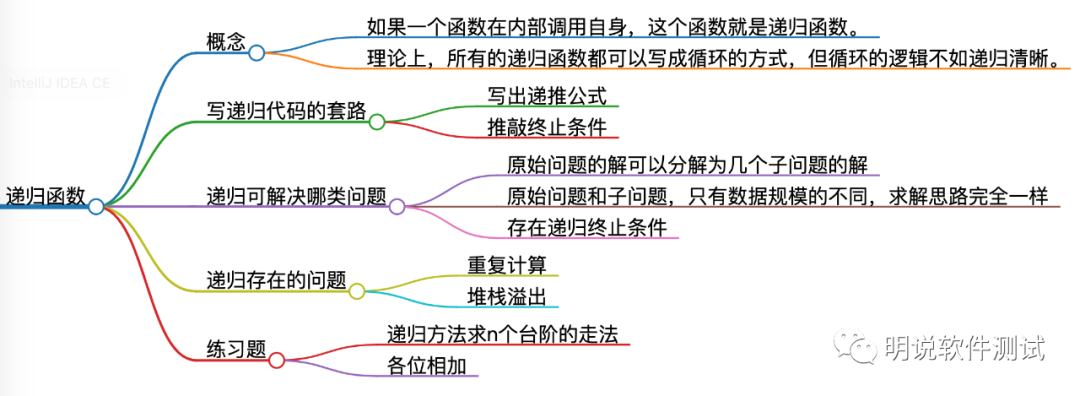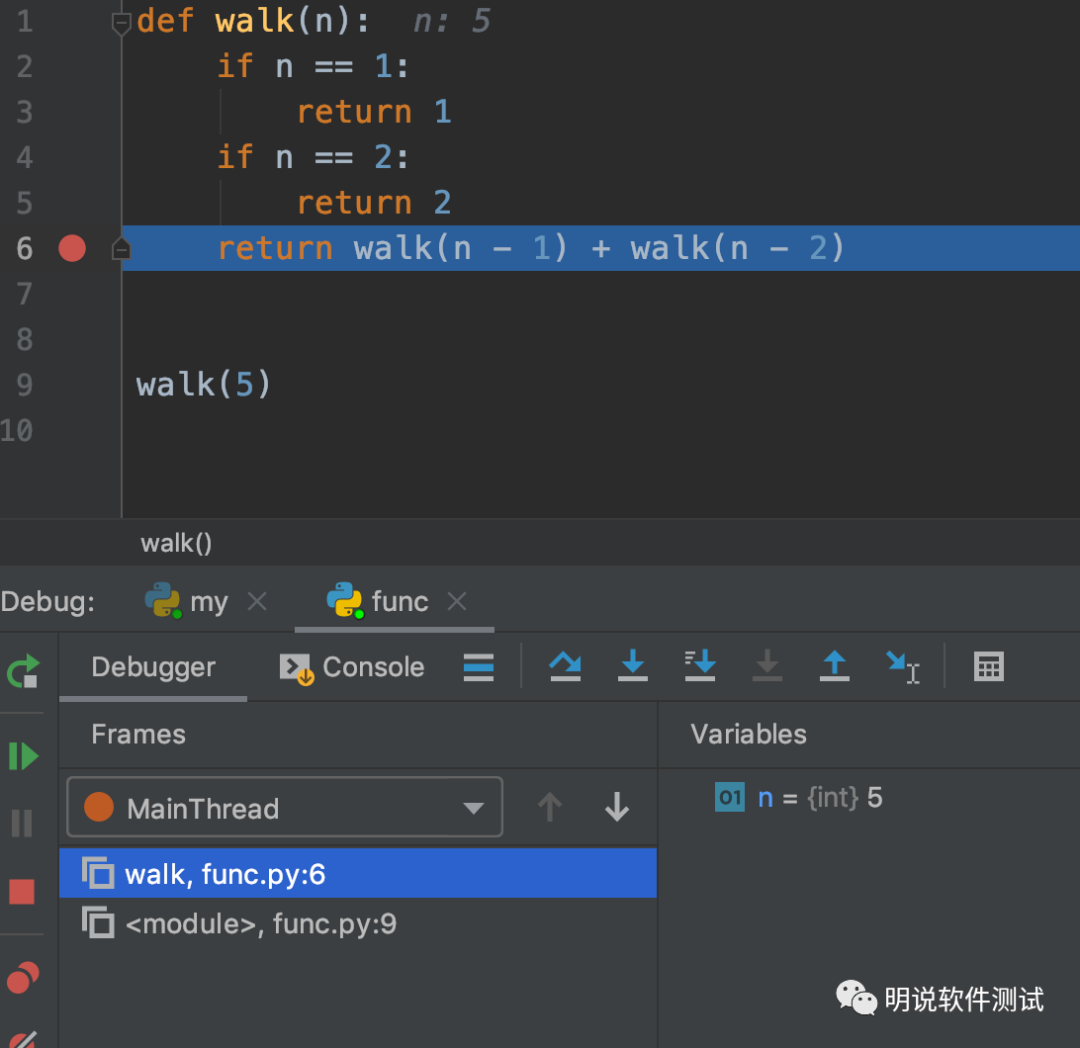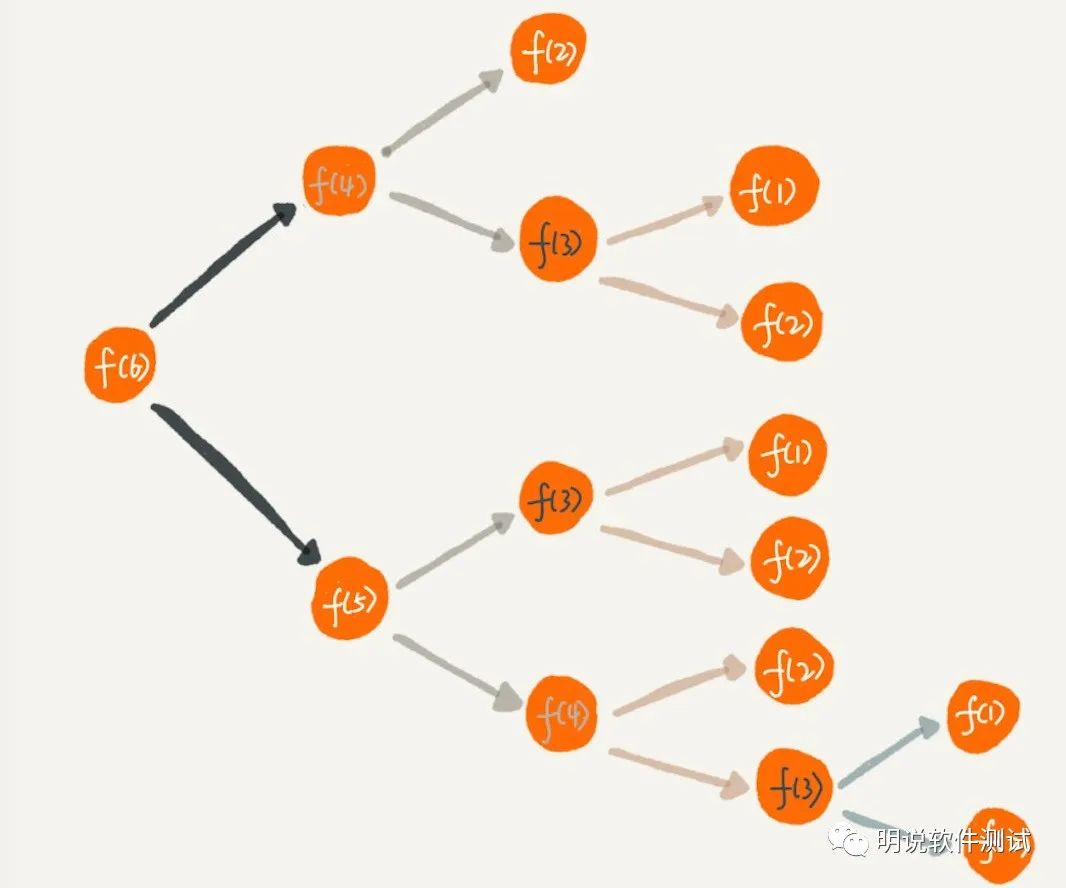## 连载|想用Python做自动化测试？递归函数 原荐

递归函数就是函数内部调用自身，可以使代码逻辑更加易懂。但是递归也有坑，需要避免。## 13.1 概念

• 在函数内部，可以调用其他函数。如果一个函数在内部调用自身，这个函数就是递归函数。

• 理论上，所有的递归函数都可以写成循环的方式，但循环的逻辑不如递归清晰。

``def fact(n):``  if n==1:``      return 1``  return n * fact(n - 1)``

## 13.2 写递归代码的套路

• 第一步，写出递推公式

• 第二步，推敲终止条件

### 13.2.1 斐波那契数列

0，1，1，2，3，5，8，13，21，34，55，…。

fibonacci(n) = fibonacci(n - 1) + fibonacci(n - 2)

``if n < 1:  # 递归终止条件``   return 0``if n in [1, 2]:  # 递归终止条件``   return 1``

``def fibonacci(n):``    if n < 1:  # 递归终止条件``        return 0``    if n in [1, 2]:  # 递归终止条件``        return 1 ``    return fibonacci(n - 1) + fibonacci(n - 2)  # 递归公式``

### 13.2.2 n 个台阶有多少种走法

``def walk(n):``    if n == 1:  # 递归终止条件``        return 1``    if n == 2:  # 递归终止条件``        return 2``    return walk(n - 1) + walk(n - 2)  # 递归公式``

## 13.3 递归可解决哪类问题

• 原始问题的解可以分解为几个子问题的解

• 原始问题和子问题，只有数据规模的不同，求解思路完全一样

• 存在递归终止条件

## 13.4 递归存在的问题

• 堆栈溢出

• 重复计算``def walk(n):``    if n == 1:``        return 1``    if n == 2:``        return 2``    if n > 100:``        raise RecursionError("recursion depth exceede 100")``    return walk(n - 1) + walk(n - 2)````data = dict()  # 保存中间结果``def walk(n):``    if n == 1:``        return 1``    if n == 2:``        return 2``    if n > 100:``        raise RecursionError("recursion depth exceed 100")``    if n in data:  # 如果在中间结果中，则直接返回，不用进入递推公式再次计算``        return data[n]``    result = walk(n - 1) + walk(n - 2)  # 在递归公式前面增加个查找步骤``    data[n] = result  # 将计算结果保存在中间结果data字典中``    return result``print(walk(6))``### 明说软件测试

2013/11/03
3.4K
6

jianglibo
2014/04/03
3.9K
3
SQLServer实现split分割字符串到列

cwalet
2014/05/21
9.6K
0
beego API开发以及自动化文档

beego API开发以及自动化文档 beego1.3版本已经在上个星期发布了，但是还是有很多人不了解如何来进行开发，也是在一步一步的测试中开发，期间QQ群里面很多人都问我如何开发，我的业余时间实在...

astaxie
2014/06/25
2.7W
22

2013/11/09
5.7K
8

01/25
0
0

kunjian

0
0
R语言入门系列之一

SYSU星空
2019/02/17
0
0
Istio-本地运行

22分钟前
9
0

2019/10/29
0
0